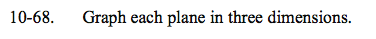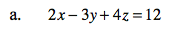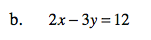Home > CCA2 > Chapter Ch10 > Lesson 10.1.4 > Problem10-68

10-68.
1. Graph each plane in three dimensions. Homework Help ✎

1. 2x − 3y + 4z = 12

2. 2x − 3y = 12Solve for each intercept. You can do this by substituting 0 for two of the variables and solving for the remaining variable.

2x − 3(0) + 4(0) = 12
2x = 12
x = 6
(6, 0, 0)

2(0) − 3y + 4(0) = 12
−3y = 12
y = −4
(0, -4, 0)

2(0) − 3(0) + 4z = 12
4z = 12
z = 3
(0, 0, 3)

Now that you know the x-, y-, and z-intercepts, plot the points, connect them, and shade them to look like a plane.Solve for the intercepts like you did in part (a).
Note that there is no z-intercept.